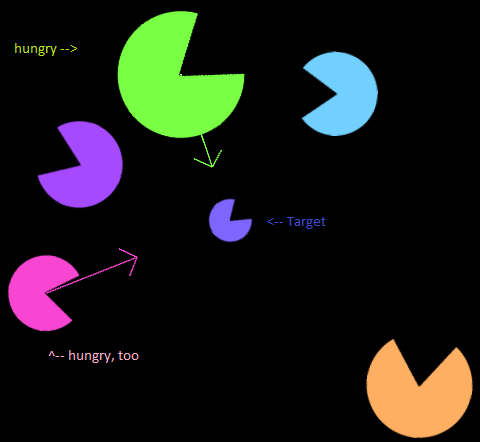# Rotate towards target in 2D space using AddTorque

Hi everybody,

I’m working on a project where pacman-like creatures chase and eat and also flee from each other.
The rotation maths give me headaches, though. I’m stuck for days, out of google keywords, found no answer which suits my problem. Though, in theory, it’s pretty simple I guess.

The situation:

2D-space, using the XY-plane.
The forward direction of my pacmans is their transform.right. (it would be nice to use transform.forward instead of .right, but that would make them move away from the camera, in z-direction, leaving their x-y-plane.)

They have sensors looking for smaller pacmans to eat. Once a sensor locks on a target we can access the target’s transform, especially it’s transform.position.

So I know the position of A and the look-direction of A. I know the position of B (its rotation does not matter).
I want A to move towards B. But only if the angle from faceDirection to targetDirection is small. If it’s too big, A shall not move but rotate so that this angle decreases.

I’d like to use the Rigidbody2D of A with .AddTorque(force) to accomplish that. In the hope of a smooth rotation without overshooting I intend to use big forces for big angles and small forces for small angles.

So bottom line I need:

• the angle to determine if A can actually move towards B or if it has to rotate first
• the proper force (positive or negative) to turn A in the right direction.

I can move and rotate my colorful fellows, I simply can’t do it right. They turn in pointless directions and run into nothingness, so I guess my math was wrong, or I fail to understand Quaternions. Can someone do it right?

In the picture below you can see a green and pink pacman who have locked on the target in the middle, indicated by the arrow gizmos. The facing direction is where the mouth is, so the green one is supposed to turn downwards while the pink one should turn up a little before moving in.I think I solved it. I created a test scene with some debug output:

As you can see, the angle is properly calculated, including the sign.

After a few seconds, it looks like this (pacmans on the left side are still turning):

And here is the code:

``````	void Update () {
// rotate if angle is bigger than that to decrease it
float maxAngle = 10f;

// where is the target?
Vector2 targetDirection = Small.transform.position - Big.transform.position;
// where are we looking?
Vector2 lookDirection = Big.transform.right;

// to indicate the sign of the (otherwise positive 0 .. 180 deg) angle
Vector3 cross = Vector3.Cross(targetDirection, lookDirection);
// actually get the sign (either 1 or -1)
float sign = Mathf.Sign(cross.z);

// the angle, ranging from 0 to 180 degrees
float angle = Vector2.Angle(targetDirection, lookDirection);

// apply the sign to get angles ranging from -180 to 0 to +180 degrees
angle *= sign;
// debug output
Text.text = angle.ToString();

// apply torque in the opposite direction to decrease angle